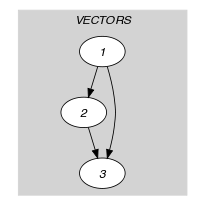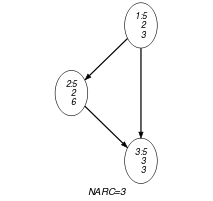## 5.220. lex_alldifferent

Origin

J. Pearson

Constraint

$\mathrm{𝚕𝚎𝚡}_\mathrm{𝚊𝚕𝚕𝚍𝚒𝚏𝚏𝚎𝚛𝚎𝚗𝚝}\left(\mathrm{𝚅𝙴𝙲𝚃𝙾𝚁𝚂}\right)$

Synonyms

$\mathrm{𝚕𝚎𝚡}_\mathrm{𝚊𝚕𝚕𝚍𝚒𝚏𝚏}$, $\mathrm{𝚕𝚎𝚡}_\mathrm{𝚊𝚕𝚕𝚍𝚒𝚜𝚝𝚒𝚗𝚌𝚝}$, $\mathrm{𝚊𝚕𝚕𝚍𝚒𝚏𝚏}_\mathrm{𝚘𝚗}_\mathrm{𝚝𝚞𝚙𝚕𝚎𝚜}$, $\mathrm{𝚊𝚕𝚕𝚍𝚒𝚏𝚏𝚎𝚛𝚎𝚗𝚝}_\mathrm{𝚘𝚗}_\mathrm{𝚝𝚞𝚙𝚕𝚎𝚜}$, $\mathrm{𝚊𝚕𝚕𝚍𝚒𝚜𝚝𝚒𝚗𝚌𝚝}_\mathrm{𝚘𝚗}_\mathrm{𝚝𝚞𝚙𝚕𝚎𝚜}$.

Type
 $\mathrm{𝚅𝙴𝙲𝚃𝙾𝚁}$ $\mathrm{𝚌𝚘𝚕𝚕𝚎𝚌𝚝𝚒𝚘𝚗}\left(\mathrm{𝚟𝚊𝚛}-\mathrm{𝚍𝚟𝚊𝚛}\right)$
Argument
 $\mathrm{𝚅𝙴𝙲𝚃𝙾𝚁𝚂}$ $\mathrm{𝚌𝚘𝚕𝚕𝚎𝚌𝚝𝚒𝚘𝚗}\left(\mathrm{𝚟𝚎𝚌}-\mathrm{𝚅𝙴𝙲𝚃𝙾𝚁}\right)$
Restrictions
 $|\mathrm{𝚅𝙴𝙲𝚃𝙾𝚁}|\ge 1$ $\mathrm{𝚛𝚎𝚚𝚞𝚒𝚛𝚎𝚍}$$\left(\mathrm{𝚅𝙴𝙲𝚃𝙾𝚁},\mathrm{𝚟𝚊𝚛}\right)$ $\mathrm{𝚛𝚎𝚚𝚞𝚒𝚛𝚎𝚍}$$\left(\mathrm{𝚅𝙴𝙲𝚃𝙾𝚁𝚂},\mathrm{𝚟𝚎𝚌}\right)$ $\mathrm{𝚜𝚊𝚖𝚎}_\mathrm{𝚜𝚒𝚣𝚎}$$\left(\mathrm{𝚅𝙴𝙲𝚃𝙾𝚁𝚂},\mathrm{𝚟𝚎𝚌}\right)$
Purpose

All the vectors of the collection $\mathrm{𝚅𝙴𝙲𝚃𝙾𝚁𝚂}$ are distinct. Two vectors $\left({u}_{1},{u}_{2},\cdots ,{u}_{n}\right)$ and $\left({v}_{1},{v}_{2},\cdots ,{v}_{n}\right)$ are distinct if and only if there exists $i\in \left[1,n\right]$ such that ${u}_{i}\ne {v}_{i}$.

Example
$\left(〈\mathrm{𝚟𝚎𝚌}-〈5,2,3〉,\mathrm{𝚟𝚎𝚌}-〈5,2,6〉,\mathrm{𝚟𝚎𝚌}-〈5,3,3〉〉\right)$

The $\mathrm{𝚕𝚎𝚡}_\mathrm{𝚊𝚕𝚕𝚍𝚒𝚏𝚏𝚎𝚛𝚎𝚗𝚝}$ constraint holds since:

• The first vector $〈5,2,3〉$ and the second vector $〈5,2,6〉$ of the $\mathrm{𝚅𝙴𝙲𝚃𝙾𝚁𝚂}$ collection differ in their third component (i.e., $3\ne 6$).

• The first vector $〈5,2,3〉$ and the third vector $〈5,3,3〉$ of the $\mathrm{𝚅𝙴𝙲𝚃𝙾𝚁𝚂}$ collection differ in their second component (i.e., $2\ne 3$).

• The second vector $〈5,2,6〉$ and the third vector $〈5,3,3〉$ of the $\mathrm{𝚅𝙴𝙲𝚃𝙾𝚁𝚂}$ collection differ in their second and third components (i.e., $2\ne 3$ and $6\ne 3$).

Typical
 $|\mathrm{𝚅𝙴𝙲𝚃𝙾𝚁}|>1$ $|\mathrm{𝚅𝙴𝙲𝚃𝙾𝚁𝚂}|>1$
Symmetries
• Items of $\mathrm{𝚅𝙴𝙲𝚃𝙾𝚁𝚂}$ are permutable.

• Items of $\mathrm{𝚅𝙴𝙲𝚃𝙾𝚁𝚂}.\mathrm{𝚟𝚎𝚌}$ are permutable (same permutation used).

• All occurrences of two distinct tuples of values of $\mathrm{𝚅𝙴𝙲𝚃𝙾𝚁𝚂}.\mathrm{𝚟𝚎𝚌}$ can be swapped; all occurrences of a tuple of values of $\mathrm{𝚅𝙴𝙲𝚃𝙾𝚁𝚂}.\mathrm{𝚟𝚎𝚌}$ can be renamed to any unused tuple of values.

Arg. properties
• Contractible wrt. $\mathrm{𝚅𝙴𝙲𝚃𝙾𝚁𝚂}$.

• Extensible wrt. $\mathrm{𝚅𝙴𝙲𝚃𝙾𝚁𝚂}.\mathrm{𝚟𝚎𝚌}$ (add items at same position).

Usage

When the vectors have two components, the $\mathrm{𝚕𝚎𝚡}_\mathrm{𝚊𝚕𝚕𝚍𝚒𝚏𝚏𝚎𝚛𝚎𝚗𝚝}$ constraint allows to directly enforce difference constraints between pairs of variables. Such difference constraints occur for instance in block design problems (e.g., Steiner triples, Kirkman schoolgirls problem). However, in all these problems a same variable may occur in more than one pair of variables. Consequently, arc-consistency is not achieved any more by the filtering algorithm described in [QuimperWalsh05].

Algorithm

A filtering algorithm achieving arc-consistency for the $\mathrm{𝚕𝚎𝚡}_\mathrm{𝚊𝚕𝚕𝚍𝚒𝚏𝚏𝚎𝚛𝚎𝚗𝚝}$ constraint is proposed by C.-G. Quimper and T. Walsh in [QuimperWalsh05] and a longer version is available in [QuimperWalshReport05] and in [QuimperWalsh06].

Reformulation

The $\mathrm{𝚕𝚎𝚡}_\mathrm{𝚊𝚕𝚕𝚍𝚒𝚏𝚏𝚎𝚛𝚎𝚗𝚝}$$\left(\mathrm{𝚅𝙴𝙲𝚃𝙾𝚁𝚂}\right)$ constraint can be expressed as a clique of $\mathrm{𝚕𝚎𝚡}_\mathrm{𝚍𝚒𝚏𝚏𝚎𝚛𝚎𝚗𝚝}$ constraints. By associating a $n$-dimensional box for which all sizes are equal to 1, one can also express the $\mathrm{𝚕𝚎𝚡}_\mathrm{𝚊𝚕𝚕𝚍𝚒𝚏𝚏𝚎𝚛𝚎𝚗𝚝}$$\left(\mathrm{𝚅𝙴𝙲𝚃𝙾𝚁𝚂}\right)$ constraint as a $\mathrm{𝚍𝚒𝚏𝚏𝚗}$ or a $\mathrm{𝚐𝚎𝚘𝚜𝚝}$ constraint. Enforcing all the $n$-dimensional boxes to not overlap is equivalent as enforcing all the vectors to be distinct. In the context of the multidimensional sweep algorithm of the $\mathrm{𝚐𝚎𝚘𝚜𝚝}$ constraint [BeldiceanuCarlssonPoderSadekTruchet07], it makes more sense to make a complete sweep over the domain of each variable in order not to only restrict the minimum and maximum value of each variable.

generalisation: $\mathrm{𝚍𝚒𝚏𝚏𝚗}$ ($\mathrm{𝚟𝚎𝚌𝚝𝚘𝚛}$ replaced by $\mathrm{𝚘𝚛𝚝𝚑𝚘𝚝𝚘𝚙𝚎}$), $\mathrm{𝚐𝚎𝚘𝚜𝚝}$ ($\mathrm{𝚟𝚎𝚌𝚝𝚘𝚛}$ replaced by $\mathrm{𝚘𝚋𝚓𝚎𝚌𝚝}$).

specialisation: $\mathrm{𝚊𝚕𝚕𝚍𝚒𝚏𝚏𝚎𝚛𝚎𝚗𝚝}$ ($\mathrm{𝚟𝚎𝚌𝚝𝚘𝚛}$ replaced by $\mathrm{𝚟𝚊𝚛𝚒𝚊𝚋𝚕𝚎}$).

Keywords
Arc input(s)

$\mathrm{𝚅𝙴𝙲𝚃𝙾𝚁𝚂}$

Arc generator
$\mathrm{𝐶𝐿𝐼𝑄𝑈𝐸}$$\left(<\right)↦\mathrm{𝚌𝚘𝚕𝚕𝚎𝚌𝚝𝚒𝚘𝚗}\left(\mathrm{𝚟𝚎𝚌𝚝𝚘𝚛𝚜}\mathtt{1},\mathrm{𝚟𝚎𝚌𝚝𝚘𝚛𝚜}\mathtt{2}\right)$

Arc arity
Arc constraint(s)
$\mathrm{𝚕𝚎𝚡}_\mathrm{𝚍𝚒𝚏𝚏𝚎𝚛𝚎𝚗𝚝}$$\left(\mathrm{𝚟𝚎𝚌𝚝𝚘𝚛𝚜}\mathtt{1}.\mathrm{𝚟𝚎𝚌},\mathrm{𝚟𝚎𝚌𝚝𝚘𝚛𝚜}\mathtt{2}.\mathrm{𝚟𝚎𝚌}\right)$
Graph property(ies)
$\mathrm{𝐍𝐀𝐑𝐂}$$=|\mathrm{𝚅𝙴𝙲𝚃𝙾𝚁𝚂}|*\left(|\mathrm{𝚅𝙴𝙲𝚃𝙾𝚁𝚂}|-1\right)/2$

Graph model

Parts (A) and (B) of Figure 5.220.1 respectively show the initial and final graph associated with the Example slot. Since we use the $\mathrm{𝐍𝐀𝐑𝐂}$ graph property, the arcs of the final graph are stressed in bold.

##### Figure 5.220.1. Initial and final graph of the $\mathrm{𝚕𝚎𝚡}_\mathrm{𝚊𝚕𝚕𝚍𝚒𝚏𝚏𝚎𝚛𝚎𝚗𝚝}$ constraint(a) (b)
Signature

Since we use the $\mathrm{𝐶𝐿𝐼𝑄𝑈𝐸}\left(<\right)$ arc generator on the $\mathrm{𝚅𝙴𝙲𝚃𝙾𝚁𝚂}$ collection the number of arcs of the initial graph is equal to $|\mathrm{𝚅𝙴𝙲𝚃𝙾𝚁𝚂}|·\left(|\mathrm{𝚅𝙴𝙲𝚃𝙾𝚁𝚂}|-1\right)/2$. For this reason we can rewrite $\mathrm{𝐍𝐀𝐑𝐂}=|\mathrm{𝚅𝙴𝙲𝚃𝙾𝚁𝚂}|·\left(|\mathrm{𝚅𝙴𝙲𝚃𝙾𝚁𝚂}|-1\right)/2$ to $\mathrm{𝐍𝐀𝐑𝐂}\ge |\mathrm{𝚅𝙴𝙲𝚃𝙾𝚁𝚂}|·\left(|\mathrm{𝚅𝙴𝙲𝚃𝙾𝚁𝚂}|-1\right)/2$ and simplify $\underline{\overline{\mathrm{𝐍𝐀𝐑𝐂}}}$ to $\overline{\mathrm{𝐍𝐀𝐑𝐂}}$.